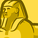# QlikView App Dev

Discussion Board for collaboration related to QlikView App Development.

cancel
Showing results for
Did you mean:Specialist

## set analysis

Hi all,

I have declared an variable

called

vMin=date(min([Comp Date]),'MM/DD/YYYY')

vMax=date(max([Comp Date]),'MM/DD/YYYY')

wheni make set analysis calucaltion for the min and max

its not showing the correcte count below is my expression i tried three ways but not getting the correct result

=sum({<[Comp Date]={'\$(vMax)'}>}[USD Medium])

=sum({<[Comp Date]=\$(vMax)>}[USD Medium])

=sum({<[Comp Date]={'=\$(vMax)'}>}[ Medium])

=sum({<[Comp Date]={"=\$(vMax)"}>}[USD Medium])

similar to min date

let me know know solution for my issue thx

7 RepliesMVP

try this

for all

=sum({<[Comp Date]={\$(=vMax)}>}[USD Medium])Specialist III

Try with :

=sum({<[Comp Date]={"=\$(=vMax)"}>}[USD Medium])Specialist
Author

not working getting over all sum of usd mediumSpecialist
Author

not working getting errorMVP

Could you share a sample qvw?MVP

it should work what error your getting could you please share the screen shot of it?MVP

is this?

add    "=" symbol before the variable while creating

=sum({<[Comp Date]={\$(=vMax)}>}[USD Medium])